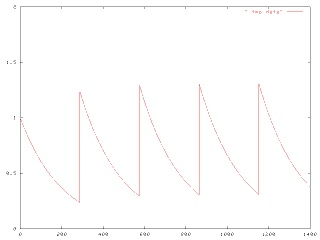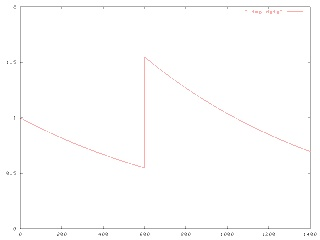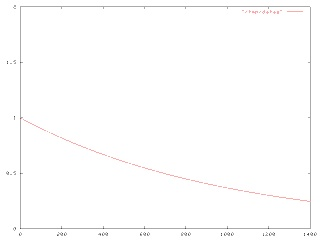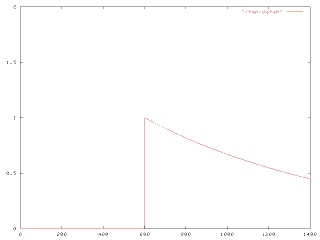# The Universe of Discourse

Thu, 30 Nov 2006

Trivial calculations
Back in September, I wrote about how I tend to plunge ahead with straightforward calculations whenever possible, grinding through the algebra, ignoring the clever shortcut. I'll go back and look for the shortcut, but only if the hog-slaughtering approach doesn't get me what I want. This is often an advantage in computer programming, and often a disadvantage in mathematics.

This occasionally puts me in the position of feeling like a complete ass, because I will grind through some big calculation to reach a simple answer which, in hindsight, is completely obvious.

One early instance of this that I remember occurred more than twenty years ago when a friend of mine asked me how many spins of a slot machine would be required before you could expect to hit the jackpot; assume that the machine has three wheels, each of which displays one of twenty symbols, so the chance of hitting the jackpot on any particular spin is 1/8,000. The easy argument goes like this: since the expected number of jackpots per spin is 1/8,000, and expectations are additive, 8,000 spins are required to get the expected number of jackpots to 1, and this is in fact the answer.

But as a young teenager, I did the calculation the long way. The chance of getting a jackpot in one spin is 1/8000. The chance of getting one in exactly two spins is (1 - 1/8000)·(1/8000). The chance of getting one in exactly three spins is (1 - 1/8000)2·(1/8000). And so on; so you sum the infinite series:

$$\sum_{i=1}^\infty {i{\left({k-1\over k}\right)^{i-1}} {1\over k}}$$

And if you do it right, you do indeed get exactly k. Well, I was young, and I didn't know any better.

I'd like to be able say I never forgot this. I wish it were true. I did remember it a lot of the time. But I can think of a couple of times when I forgot, and then felt like an ass when I did the problem the long way.

One time was in 1996. There is a statistic in baseball called the on-base percentage or "OBP"—please don't all go to sleep at once. This statistic measures, for each player, the fraction of his plate appearances in which he is safe on base, typically by getting a hit, or by being walked. It is typically around 1/3; exceptional players have an OBP as high as 2/5 or even higher. You can also talk about the OBP of a team as a whole.

A high OBP is a very important determiner of the number of runs a baseball team will score, and therefore of how many games it will win. Players with a higher OBP are more likely to reach base, and when a batter reaches base, another batter comes to the plate. Teams with a high overall OBP therefore tend to bring more batters to the plate and so have more chances to score runs, and so do tend to score runs, than teams with a low overall OBP.

I wanted to calculate the relationship between team OBP and the expected number of batters coming to the plate each inning. I made the simplifying assumption that every batter on the team had an OBP of p, and calculated the expected number of batters per inning. After a lot of algebra, I had the answer: 3/(1-p). Which makes perfect sense: There are only two possible outcomes for a batter in each plate appearance: he can reach base, or he can be put out; these are exclusive. A batter who reaches base p of the time is put out 1-p of the time, consuming, on average, 1-p of an out. A team gets three outs in an inning; the three outs are therefore consumed after 3/(1-p) batters. Duh.

This isn't the only baseball-related mistake I've made. I once took a whole season's statistics and wrote a program to calculate the average number of innings each pitcher pitched. Okay, no problem yet. But then I realized that the average was being depressed by short relievers; I really wanted the average only for starting pitchers. But how to distinguish starting pitchers from relievers? Simple: the statistics record the number of starts for each pitcher, and relievers never start games. But then I got too clever. I decided to weight each pitcher's contribution to the average by his number of starts. Since relievers never start, this ignores relievers; it allows pitchers who do start a lot of games to influence the result more than those who only pitched a few times. I reworked the program to calculate the average number of innings pitched per start.

The answer was 9. (Not exactly, but very close.)

It was obviously 9. There was no other possible answer. There is exactly one start per game, and there are 9 innings per game,1 and some pitcher pitches in every inning, so the number of innings pitched per start is 9. Duh.

Anyway, the real point of this article is to describe a more sophisticated mistake of the same general sort that I made a couple of weeks ago. I was tinkering around with a problem in pharmacology. Suppose Joe has some snakeoleum pills, and is supposed to take one capsule every day. He would like to increase the dosage to 1.5 pills every day, but he cannot divide the capsules. On what schedule should he take the pills to get an effect as close as possible to the effect of 1.5 pills daily?

I assumed that we could model the amount of snakeoleum in Joe's body as a function f(t), which normally decayed exponentially, following f(t) = ae-kt for some constant k that expresses the rate at which Joe's body metabolizes and excretes the snakeoleum. Every so often, Joe takes a pill, and at these times d0, d1, etc., the function f is discontinuous, jumping up by 1. The value a here is the amount of snakeoleum in Joe's body at time t=0. If Joe takes one pill every day, the (maximum) amount of snakeoleum in his body will tend toward 1/(1-e-k) over time, as in the graph below:I wanted to compare this with what happens when Joe takes the pill on various other schedules. For some reason I decided it would be a good idea to add up the total amount of pill-minutes for a particular dosage schedule, by integrating f. That is, I was calculating !!\int_a^b f(t) dt!! for various a and b; for want of better values, I calculated the total amount !!\int_0^\infty f(t) dt!!.

Doing this for the case in which Joe takes a single pill at time 0 is simple; it's just !!\int_0^\infty e^{-kt} dt!!, which is simply 1/k.

But then I wanted to calculate what happens when Joe takes a second pill, say at time M. At time M, the amount of snakeoleum left in Joe's body from the first pill is e-kM, so the function f has f(t) = e-kt for 0 ≤ tM and f(t) = (e-kM+1)e-k(t-M) for Mt < ∞. The graph looks like this:After the second pill, at t=600, it decays gradually to zero. I wanted to integrate this function. Since it's continuous on the two pieces of the interval, we break the integral into two:

\halign{ \hfil# & = # \hfil  & + #\hfil \cr \int_0^\infty f(t) dt & \int_0^M f(t) dt & \int_M^\infty f(t) dt \cr & \int_0^M e^{-kt} dt & \int_M^\infty (e^{-kM} + 1)e^{-k(t-M)} dt \cr & {1 \over k }(1-e^{-kM}) & {1\over k}(e^{-kM} + 1)\cr & \ldots & \omit \cr }.

Well, you get the idea. The answer was 2/k.

In retrospect, this is completely obvious.

This is because of the way I modeled the pills. When the decay of f(t) is exponential, as it is here, that means that the rate at which the snakeoleum is metabolized is proportional to the amount: twice as much snakeoleum means that the decay is twice as fast. or, looked at another way, each pill decays independently of the rest. Put two pills in, and an hour later you'll find that you have twice as much left as if you had only put one pill in.

Since the two pills are acting independently, you can calculate their effect independently. That is, f(t) can be decomposed into f1(t) + f2(t), where f1 is the contribution from the first pill:And f2 is the contribution from the second pill:But the second pill is exactly like the first pill. The only difference is that Joe takes it a little later. But since the integrals are considering the total effect from now to eternity, the time at the beginning is unimportant, and the effect of the second pill might as well be:Just shift your head over a bit. (Mathematically, this is equivalent to substituting y = x-M in the second integral.) So of course it is the same as the first pill, and the result is exactly 2/k. When I saw the 2/k, I scratched my head for a minute, then figured it out and said "duh".

Anyway, I think what I really want is to find !!\int_0^\infty {\left(f_1(t) - f_{1.5}(t, d)\right)}^2 dt!!, where f1 is the function that describes the amount of snakeoleum when Joe takes one pill a day, and f1.5 is the function that describes the amount when Joe takes 1.5 pills every d days. But if there's one thing I think you should learn from a dumbass mistake like this, it's that it's time to step back and try to consider the larger picture for a while, so I've decided to do that before I go on.